-=+=- -=+=- -=+=- -=+=- -=+=- -=+=- -=+=- -=+=- -=+=- -=+=- -=+=- -=+=- -=+=- -=+=- -=+=- -=+=- -=+=- -=+=- -=+=- -=+=- -=+=- -=+=- -=+=- -=+=- -=+=- -=+=- -=+=- -=+=- -=+=- -=+=- (c) WidthPadding Industries 1987 0|342|0 -=+=- -=+=- -=+=- -=+=- -=+=- -=+=- -=+=- -=+=- -=+=- -=+=- -=+=- -=+=- -=+=- -=+=- -=+=- -=+=- -=+=- -=+=- -=+=- -=+=- -=+=- -=+=- -=+=- -=+=- -=+=- -=+=- -=+=- -=+=- -=+=- -=+=-

### Welcome to Socoder

Socoder is a community of coders, who sit in a quiet corner of the internet, chatting endlessly about random nonsense.
Occasionally we code, too.

If you'd like to join in with the chatter, feel free to register.

On the top left is a little cog which you can use to open the Settings sidebar, where you'll find the registration and login areas. Once you're logged in, this is also where most of the menu-based things will appear.
On the top right is a secondary sidebar button which can be used to reveal the most recent topics. You may dock either of these sidebars into place, using the provided links.
As well as these, there's a paintbrush on the top right to help you style the site using a variety of themes and other such settings.

Tricky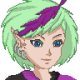# Kthura - Map Editor

24th October 2019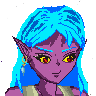Let's show you my Kthura Map Editor

[img]https://user-images.githubusercontent.com/11202073/67527190-b6087880-f6b6-11e9-8fdd-c2a2b47011b3.png[/img]

Kthura is a map system for 2D Map development, but here's the rub... It's not a tile s*snip*

Tricky# Astrobounce

18th October 2019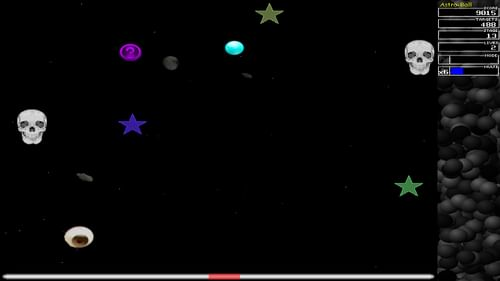Astrobounce

HoboBen# Pyrrhic: programmable python build tool

24th September 2019Pyrrhic is a programmable Python build system that supports incremental compilation, dynamic dependencies, and builtins for a range of tasks.

Pyrrhic is great for building static websites, or any build process where tasks are written in Python.

AndyH# Blockman Gets Twenty

24th August 2019Blockman Gets Twenty

Pakz# Pico8 inspired sprite/map editor

1st August 2019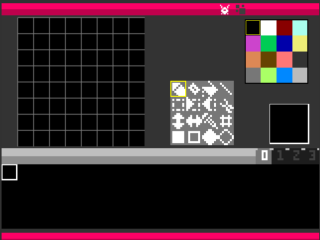pico8 inspired sprite and map editor(web / online)

therevillsgames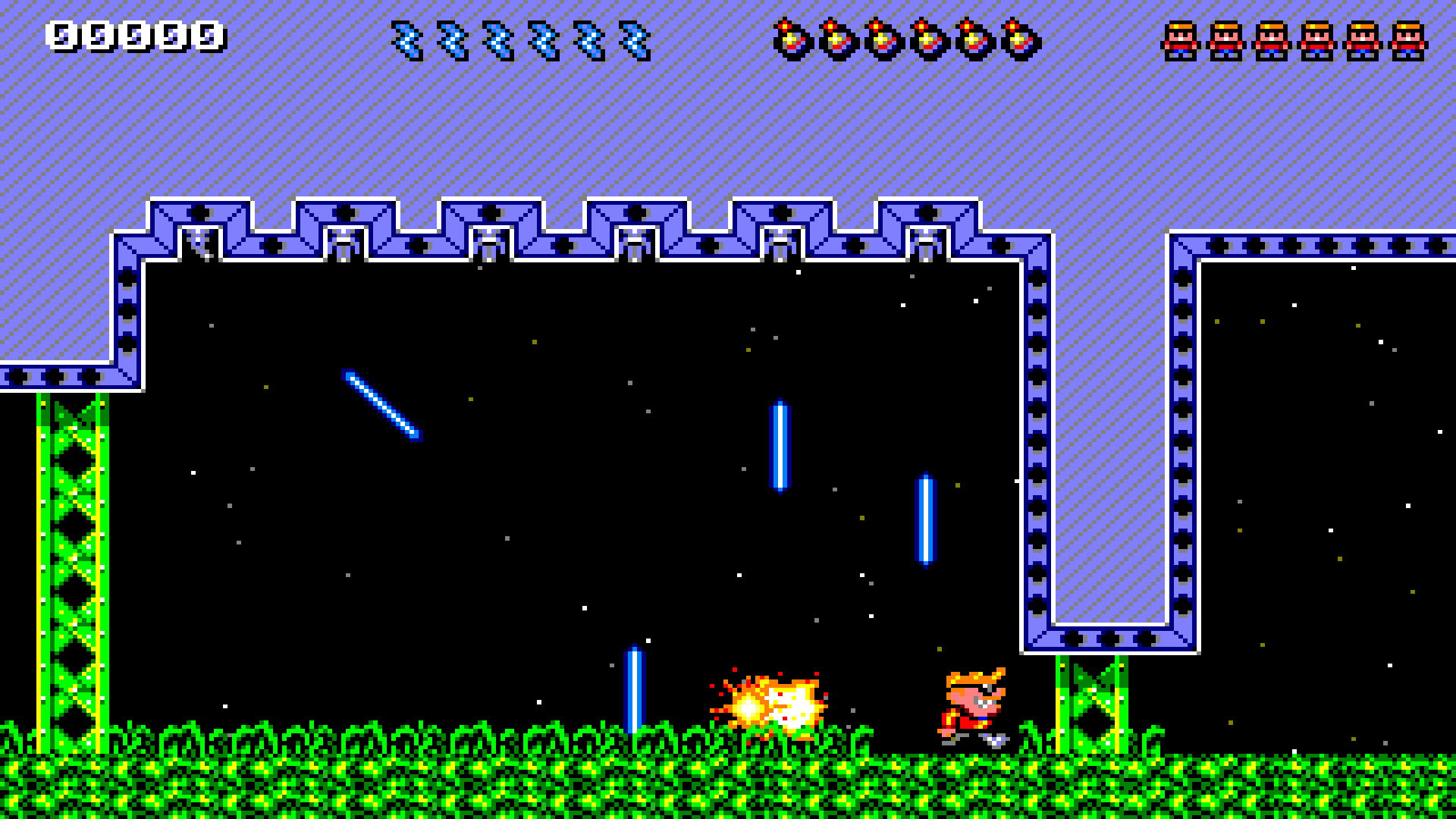When Rick dies, Jack steps up!